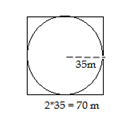# RRB ALP 2018 Practice Test Papers | Arithmetic Questions (Day-74)

Dear Aspirants, Here we have given the Important RRB ALP & Technicians Exam 2018 Practice Test Papers. Candidates those who are preparing for RRB ALP 2018 can practice these Arithmetic Questions to get more confidence to Crack RRB 2018 Examination.

[WpProQuiz 3047]

Click “Start Quiz” to attend these Questions and view Solutions

1) Neeraj’s salary increased by 30% and decreased by 30% subsequently. Find the percentage of salary increased / decreased?

a) 15 % Decreased

b) 12 % Increased

c) 9 % Decreased

d) 18 % Increased

2) Rs. 27000 divided among L, M and N in the ratio of 7:5:3. What is the difference between highest share amount and lowest share amount?

a) 6000

b) 7200

c) 5000

d) 6000

3) SamiraborrowsRs. 20000 on the rate of 12% for 3 years. What is the simple interest on that amount?

a) 7500

b) 4500

c) 7200

d) 3600

4) Simplify:

2/5 of 1/7 of 1/9 of 945 =?

a) 6

b) 4

c) 3

d) 2

5) A number is added with its 2/7th of itself gives 423. What is the 40% of that number?

a) 130

b) 131.6

c) 130.4

d) 120.6

6) 10 men can works 6 hours a day finished the work in 10 days, if the work have to finish 5 days 10 men can work how many hours a day?

a) 24 hours

b) 8 hours

c) 2 hours

d) 12 hours

7) 1111 + 100.11 + 111.01 + 0.111 =?

a) 1223. 132

b) 1322.231

c) 1212 .231

d) 1231.123

8) A boat  travels along the stream in 12kmph and return against the stream in 8 kmph. Find the speed of Boat

a) 4 kmph

b) 10kmph

c) 5kmph

d) 2kmph

9) A circular garden fencing with rectangular path. Radius of the garden is 35 m. Ratio of length and breadth is 7:5. Find the Circumference of the rectangular path.

a) 540

b) 360

c) 240

d) 120

10) Sin 30° = ?

a) 0

b) 1/2

c) 1/ √2

d) 1

Salary increase = 30%

Salary Decrease = 30%

Percentage of salary increased / decreased = x – y +  = 30 – 30 +  = 9% decreased.

Total amount = Rs. 27000

Ratio of shares = 7: 5: 3

Amount received by L = 12600;        M = 9000;       N = 5400

Difference = 12600 – 5400 = 7200 .

S.I = PNR/100

S.I = 20000×3×12/100

S.I = 7200

2/5 * 1/7 * 1/9 * 945 = 6.

X + 2x/7 = 423

(7x + 2x)/7 = 423

9x/7 = 423

X = 423*7/9 = 329

40% of 329 = 40*329/100 = 131.6

M1D1H1 = M2D2H2

10×6×10 = 10 × 5 × H

Hours = (10×6×10) / (10×5) = 60 / 5 = 12 hours per day.

Speed of Boat = (U+V)/2 = (12+8)/2 = 20/2 = 10kmph.R = 35 m

Length of rectangle = 70 m

Breathe of the rectangle = 50 m

Circumference of the rectangle = 2(70+50) 2*120 = 240 m